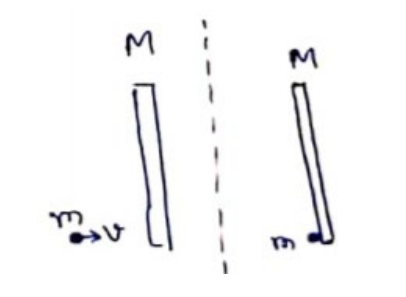# Solve the following :`
Question:

Suppose the particle of the previous problem has a mass $m$ and speed $v$ before the collision and sticks to the rod after the collision. The rod has a mass M. (a) Find the velocity of the centre of mass $\mathrm{C}$ of the system constituting "the rod plus the particle". (b) Find the velocity of the particle with respect to $C$ before the collision. (c) Find the velocity of the rod with respect to $C$ before the collision.

(d) Find the angular momentum of the particle and of the rod about the centre of mass $C$ before the collision. (e) Find the moment of inertia of the system about the vertical axis through the centre of mass $C$ after the collision. (f) Find the velocity of the centre of mass $C$ and the angular velocity of the system about the centre of mass after the collision.

Solution:(a) $F_{e x t}=0$

$P_{i}=P_{f}$

$m v=(M+m) v^{\prime}$

$V^{\prime}=\frac{m v}{(M+m)}$

(b) velocity of the particle with respect to COM

$v_{c}=\mathrm{V}-\mathrm{V}^{\prime}$

$=\mathrm{V}-\frac{m v}{(M+m)}$

$=\frac{M M}{(M+\Sigma)}$

(c) velocity of the rod wrt the centre of mass $=\frac{-m v}{(M+m)}$

(d) Distance of COM from the particle

$\chi_{c m}=\frac{M \times \frac{L}{n}+m \times 0}{M+m} \Longrightarrow \chi_{c m}=\frac{M L}{2(M+m)}$

$=\frac{m M^{2} w L}{2(M+m)^{2}}$

Angular momentum of rod about COM

$=\mathrm{M} \times \frac{M v}{(M+m)} \times \frac{1}{2} \frac{M L}{(M+m)}$

$=\frac{M m^{2} v L}{2(M+m)^{2}}$

(e) M.I. about COM $=I_{1}+I_{2}$

$\mathrm{l}=\mathrm{m}\left[\frac{M L}{2(M+m)}\right]^{2}+\frac{M L^{2}}{12}+M\left[\frac{M L}{2(M+m)}\right]^{2}$

$1=\frac{M(M+4 m) L^{3}}{12(M+m)}$

$v_{C M}=\frac{m v}{(M+m)}$
$\therefore l \omega=m v r \Rightarrow w=\frac{6 m v}{(M+4 m) L}$